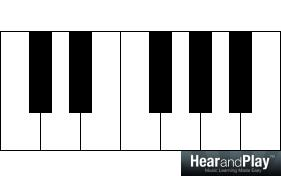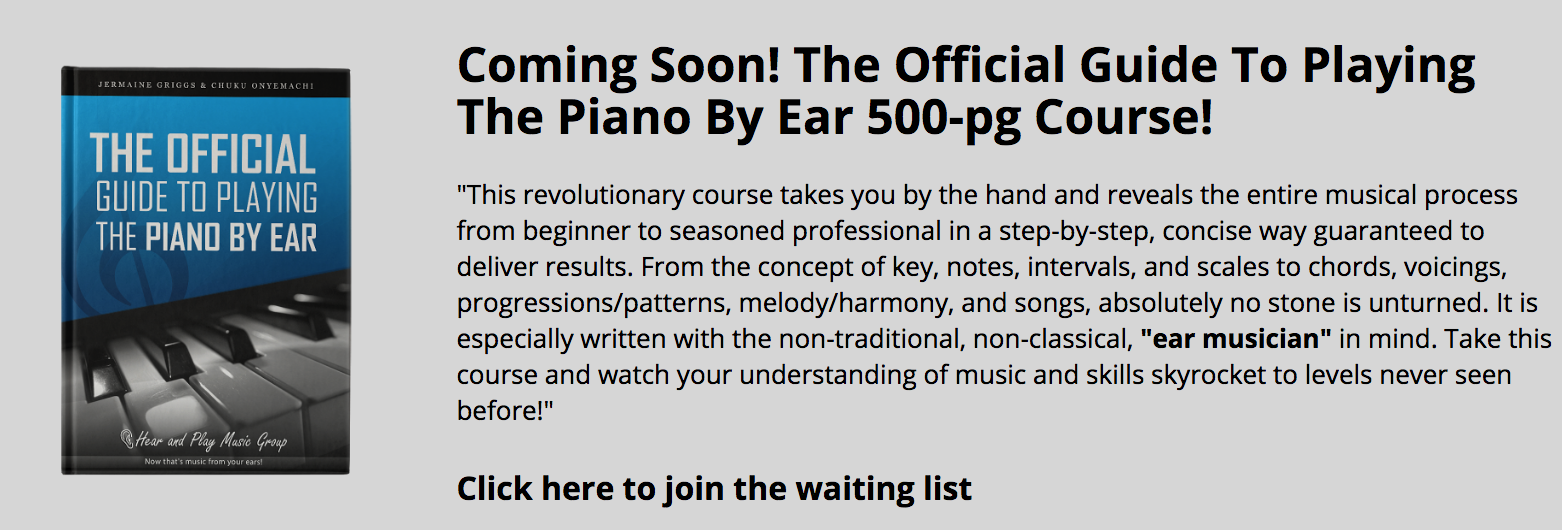• # Inversion: “What Are The Three Things That Change When An Interval Is Inverted?”In the inversion of an interval, there are three things that change:

The Direction

The Size

The Quality

…and our focus in this lesson is to highlight and explain these changes.

A great way to start is for us to refresh our minds on the inversion of intervals before we go into what we have to cover. So, if you’re coming across the concept of inversion for the first time, we got you covered.

## A Short Note On The Inversion Of An Interval

There are so many definitions of an interval from the most simple to the most scholarly. However, I’ll prefer to use this definition of an interval:

An interval is a product of the relationship between two notes (whether played or heard together or separately).

The key word that is relevant to our discourse is “two notes”.

An interval consists of two notes: the lower note and the upper note and is usually reckoned upwards. What this means is that (most of the time) the lower note of an  interval is considered before the upper note.

In the interval, C-E:C is the upper note:…while E is the lower note:“Now, Here’s How To Invert An Interval…”

An interval can be inverted by simply changing the position of the notes; the lower note would become the upper note and and upper note would become the lower note.

In the case of C-E:When inverted, C-E:…would become E-C:Submission: Every other interval can be inverted this way.

Now that we’ve refreshed our minds on the concept of inversion, let’s go into the three things that change in an interval when it is inverted.

We’ll be starting with the change of direction.

## #1 – The Change Of Direction

The direction of an interval changes when it’s inverted. Let’s take a closer look at how this works.

### “…From Upward To Downward”

The direction of an interval changes from upwards to downwards when inverted. For example, the interval “C-E” implies that C is the lower note and E is the upper note:…and this is because intervals are reckoned upwards; so, after C:…the next note (which is E):…should be placed in the upward direction.

So, the interval “C-E” (in the upward direction):…implies:

C:…and an E:…to produce C-E:The concept of inversion changes the direction from an upward direction to a downward direction. So, C-E in the downward direction is given as:

C:E (notice that the E is in the opposite direction):…and this produces E-C:…and inversion of the given interval (C-E).

So, when an interval “D-F#” is given in the upward direction:D:F#:Its direction changes to the downward direction when inverted:

D:F#:…to produce “F#-D”:…an inversion of the given interval.

### “…From Downward To Upward”

An interval can be reckoned downwards. For example, you can be asked to determine the note that is a minor third below C:A minor third above C:…is Eb:But that is NOT the note that is a minor third “below” C. Going a minor third below C is A:

C:…then a minor third below C (which is A):Altogether, that’s “A-C”:However, going by the direction, the direction of the interval is is downwards:

C:A:The inversion of “A-C”:…which is based on the downward direction produces the same interval but the upwards direction:

C:…then A in the upward direction:So, the inversion of the “A-C” interval (“C-A” in the downward direction):

C:A:…produces C-A:

C:A:Keep in mind that as regards intervals that are given in the downward direction, the second tone is played first and the first tone is played in the downward direction.

In the “A-C” interval (“C-A” in the downward direction):

C (the second tone):…is played before A (in the downward direction):## #2 – The Change Of Size

The size of an interval changes when it is inverted. Let’s take a look at what second, third, fourth, fifth, sixth, and seventh intervals become when they are inverted.

### The Inversion Of Second Intervals

The inversion of a second interval produces a seventh interval. For example, the second interval “C-D”:…becomes “D-C” (a seventh interval) when inverted:Conversely, seventh intervals change to second intervals when they are inverted.

The interval between C and B (a seventh interval):…when inverted to “B-C”:…changes to a second interval.

Submission: All second intervals become seventh intervals when inverted and vice-versa.

### The Inversion Of Third Intervals

When third intervals are inverted, they change from being third intervals into being sixth intervals. An example of a third interval is from C to E:…which becomes “E-C” (when inverted):The interval between E and C is a sixth interval. So, inverting “C-E” to “E-C” changes a third interval to a sixth interval:

C-E (third interval):E-C (sixth interval):In the same way, every other third interval can be STRICTLY inverted into a sixth interval. Also keep in mind that sixth intervals change to third intervals when inverted.

### The Inversion Of Fourth Intervals

The interval between C and F:…is a fourth interval because it encompasses four scale tones: C, D, E, and F:However, when C-F is inverted, it becomes F-C:C-F:F-C:…and F-C is a fifth interval (encompasses five scale tones: F, G, A, B, and C):So, the inversion of a fourth interval (C-F in the last example) produces a fifth interval (F-C).

Attention: It is strictly accurate that fifth intervals become fourth intervals when they are inverted.

## Final Words

Understanding the changes an interval undergoes when it’s inverted deepens your understanding of intervals.

With what we covered in this lesson, we can describe the inversion of a interval as “the change of the direction, size, and quality of an interval” and that would still be correct.

In another lesson in the not-too-distant future, I’ll be bringing you yet another deeper insight on what the inversion of a chord really is.

Until then, keep on keeping on.

All the best!

The following two tabs change content below.#### Chuku Onyemachi

Head of Education at HearandPlay Music Group
Hello, I'm Chuku Onyemachi (aka - "Dr. Pokey") - a musicologist, pianist, author, clinician and Nigerian. Inspired by my role model Jermaine Griggs, I started teaching musicians in my neighborhood in April 2005. Today, I'm privileged to work as the head of education, music consultant, and chief content creator with HearandPlay Music Group sharing my wealth of knowledge with hundreds of thousands of musicians across the world.#### Latest posts by Chuku Onyemachi (see all)